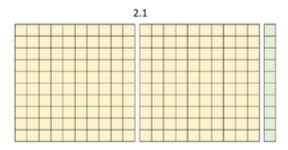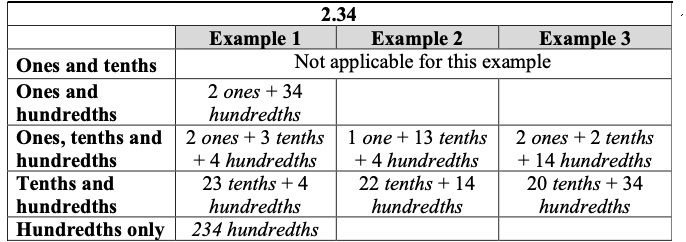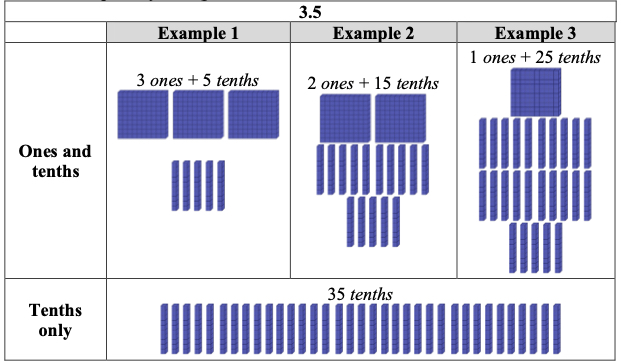# MA.5.NSO.1.3Export Print
Compose and decompose multi-digit numbers with decimals to the thousandths in multiple ways using the values of the digits in each place. Demonstrate the compositions or decompositions using objects, drawings and expressions or equations.

### Examples

The number 20.107 can be expressed as 2 tens + 1 tenth+7 thousandths or as 20 ones + 107 thousandths.
General Information
Subject Area: Mathematics (B.E.S.T.)
Strand: Number Sense and Operations
Status: State Board Approved

## Benchmark Instructional Guide

• NA

### Vertical Alignment

Previous Benchmarks

Next Benchmarks

### Purpose and Instructional Strategies

The purpose of this benchmark is for students to use place value relationships to compose and decompose multi-digit numbers with decimals. While students have composed and decomposed whole numbers in grade 3 (MA.3.NSO.1.2) and fractions in grade 4 (MA.4.FR.2.1), naming multi-digit decimals in flexible ways in grade 5 helps students with decimal comparisons and operations (addition, subtraction, multiplication and division). Flexible representations of multi- digit numbers with decimals also reinforces the understanding of how the value of digits change if they move one or more places left or right (MA.5.NSO.1.1). Composing and decomposing numbers also helps build the foundation for further work with the distributive property in grade 6 (MA.6.NSO.3.2).
• Instruction may include multiple representations using base ten models (MTR.2.1). During instruction, teachers should emphasize that the value of a base ten block (or another concrete model) is flexible (e.g., one flat could be 1 ten, one, tenth, hundredth, and so forth). Using base ten models flexibly helps students think about how numbers can be composed and decomposed in different ways.
• For example, the image below shows 2.1. This representation shows that 2.1 can also be composed as 21 tenths or 210 hundredths. Thinking about 2.1 as 210 hundredths may help subtracting 2.1 – 0.04 easier for students because they can think about the expression as 210 hundredths minus 4 hundredths, or 206 hundredths• Representing multi-digit numbers with decimals flexibly can help students reason through multiplication and division as well. For example, students may prefer to multiply 1.2 × 4 as 12 tenths × 4 to use more familiar numbers (MTR.2.1, MTR.5.1).
• Students should name their representations in different forms (e.g., word, expanded) during classroom discussion. While students are representing multi-digit numbers with decimals in different ways, teachers should invite all answers and have students compare them (MTR.4.1).

### Common Misconceptions or Errors

• Students may assume that the value of base ten blocks are fixed based on their previous experiences with whole numbers (e.g., units are ones, rods are tens, flats are hundreds). During instruction, teachers should name a base ten block for each example so students can relate the other blocks. (For example, “Show 2.4. Allow 1 rod to represent 1 tenth.”)

### Strategies to Support Tiered Instruction

• Instruction includes opportunities to decompose multi-digit numbers with decimals to the hundredths in multiple ways. Instruction includes the use of base-ten blocks to represent decimals where one flat represents one whole, one rod represents one tenth and one unit represents one hundredth. During instruction, the teacher names a base ten block for each example, so students relate the other blocks. A chart can be used to organize students’ thinking. The teacher asks students to identify the different ways to name the values (grouping the hundredths into tenths and the tenths into the ones, e.g., 2 ones and 34 hundredths or 20 tenths and 34 hundredths, etc.)
• For example, decompose 2.34 in multiple ways using ones, tenths and hundredths.• For example, show 3.5. Allow one rod to represent one-tenth. Then, decompose 3.5 in multiple ways using ones, tenths and hundredths.Using base ten blocks, show 1.36 in two different ways. Allow one flat to represent 1 whole.

How many tenths are equivalent to 13.2? How do you know?

### Instructional Items

Select all the ways to name 14.09.
• a. 1,409 hundredths
• b. 1 ten + 409 hundredths
• c. 1 ten + 4 ones + 9 tenths
• d. 140 tenths + 9 hundredths
• e. 1,409 tenths

*The strategies, tasks and items included in the B1G-M are examples and should not be considered comprehensive.

## Related Courses

This benchmark is part of these courses.
5012070: Grade Five Mathematics (Specifically in versions: 2014 - 2015, 2015 - 2022, 2022 and beyond (current))
7712060: Access Mathematics Grade 5 (Specifically in versions: 2014 - 2015, 2015 - 2018, 2018 - 2022, 2022 and beyond (current))
5012065: Grade 4 Accelerated Mathematics (Specifically in versions: 2019 - 2022, 2022 and beyond (current))
5012015: Foundational Skills in Mathematics 3-5 (Specifically in versions: 2019 - 2022, 2022 and beyond (current))

## Related Access Points

Alternate version of this benchmark for students with significant cognitive disabilities.
MA.5.NSO.1.AP.3: Compose and decompose multi-digit numbers with decimals to the hundredths. Demonstrate each composition or decomposition with objects, drawings, expressions or equations. Multi-digit numbers not to exceed 9.99.

## Related Resources

Vetted resources educators can use to teach the concepts and skills in this benchmark.

## Original Student Tutorial

Cracking the Decimal Code:

By the end of this tutorial, you will be able to read and write decimals to the thousandths using base-ten numerals, number names, and expanded form.

Type: Original Student Tutorial

## Original Student Tutorials Mathematics - Grades K-5

Cracking the Decimal Code:

By the end of this tutorial, you will be able to read and write decimals to the thousandths using base-ten numerals, number names, and expanded form.

## Student Resources

Vetted resources students can use to learn the concepts and skills in this benchmark.

## Original Student Tutorial

Cracking the Decimal Code:

By the end of this tutorial, you will be able to read and write decimals to the thousandths using base-ten numerals, number names, and expanded form.

Type: Original Student Tutorial

## Parent Resources

Vetted resources caregivers can use to help students learn the concepts and skills in this benchmark.